## GATE 2007

 Question 1

Consider the following two statements about the function f(x)=|x|

```P. f(x) is continuous for all real values of x
Q. f(x) is differentiable for all real values of x ```

Which of the following is TRUE?

 A P is true and Q is false. B P is false and Q is true. C Both P and Q are true. D Both P and Q are false.
Engineering-Mathematics       Calculus
Question 1 Explanation:
f(x) = |x|
→ f(x) is continuous for all real values of xFor every value of x, there is corresponding value of f(x).
For x is positive, f(x) is also positive
x is negative, f(x) is positive.
So, f(x) is continuous for all real values of x.
→ f(x) is not differentiable for all real values of x. For x<0, derivative is negative
x>0, derivative is positive.
Here, left derivative and right derivatives are not equal.
 Question 2

Let S be a set of n elements. The number of ordered pairs in the largest and the smallest equivalence relations on S are:

 A n and n B n2 and n C n2 and 0 D n and 1
Engineering-Mathematics       Sets-And-Relation
Question 2 Explanation:
A relation is said to be equivalent relation if it satisfies,
→ Reflexive
→ Symmetric
→ Transitive
Let a set S be,
S = {1, 2, 3}
Now, the smallest relation which is equivalence relation is,
S×S = {(1,1), (2,2), (3,3)}
= 3
= n (for set of n elements)
And, the largest relation which is equivalence relation is,
S×S = {(1,1), (1,2), (1,3), (2,1), (2,2), (2,3), (3,1), (3,2), (3,3)}
= 9
= 32
= n2 (for set of n elements)
 Question 3

What is the maximum number of different Boolean functions involving n Boolean variables?

 A n2 B 2n C 22n D 2n2
Digital-Logic-Design       Boolean-Algebra
Question 3 Explanation:
Each “boolean” variable has two possible values i.e 0 and 1.
Number of variables= n
Number of input combinations is 2n.
Each “boolean” function has two possible outputs i.e 0 and 1.
Number of boolean functions possible is 22n.
Formula: The number of m-ary functions possible with n k-ary variables is mkn.
 Question 4

Let G be the non-planar graph with the minimum possible number of edges. Then G has

 A 9 edges and 5 vertices B 9 edges and 6 vertices C 10 edges and 5 vertices D 10 edges and 6 vertices
Engineering-Mathematics       Graph-Theory
Question 4 Explanation:
Using Euler’s formula we know that,
if n ≥ 3 then e ≤ 3n-6 (for planarity)
where n = no. of vertices
e = no. of edges
Now lets check the options.
A) e=9, n=5
9 ≤ 3(5) - 6
9 ≤ 15 - 6
9 ≤ 9
Yes, it is planar.
B) e=9, n=6
9 ≤ 3(6) - 6
9 ≤ 18 - 6 9 ≤ 12 Yes, it is planar.
iii) e=10, n=5
10 ≤ 3(5) - 6
10 ≤ 15 - 6
10 ≤ 9 No, it is not planar.
So, option C is non-planar graph.
iv) e=10, n=6
10 ≤ 3(6) - 6
10 ≤ 18 - 6
10 ≤ 12
Yes, it is planar.
 Question 5

Consider the DAG with Consider V = {1, 2, 3, 4, 5, 6}, shown below. Which of the following is NOT a topological ordering?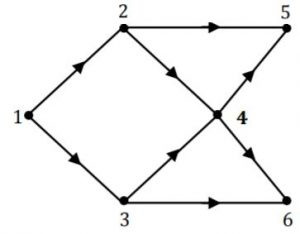A 1 2 3 4 5 6 B 1 3 2 4 5 6 C 1 3 2 4 6 5 D 3 2 4 1 6 5
Data-Structures       Graphs
Question 5 Explanation:
The process to find topological order is,
(i) Go with vertex with indegree 0.
(ii) Then remove that vertex and also remove the edges going from it.
(iii) Repeat again from (i) till every vertex is completed.
Now we can see that in option (D), '3' is given first which is not possible because indegree of vertex '3' is not zero.
Hence option (D) is not topological ordering.
 Question 6

Which of the following problems is undecidable?

 A Membership problem for CFGs. B Ambiguity problem for CFGs. C Finiteness problem for FSAs. D Equivalence problem for FSAs.
Theory-of-Computation       Decidability-and-Undecidability
Question 6 Explanation:
Whether a given CFG is ambiguous, this problem is undecidable. The reason is there is no algorithm exist for this. Remaining all are decidable.
 Question 7

Which of the following is TRUE?

 A Every subset of a regular set is regular. B Every finite subset of a non-regular set is regular. C The union of two non-regular sets is not regular. D Infinite union of finite sets is regular.
Theory-of-Computation       Regular-Language
Question 7 Explanation:
If a set is finite then it must be regular , as every language which contains finite elements is regular. Hence, every finite subset of a non-regular set is regular.
Every subset of regular set is regular, is false. For example L = {an bn | n ≥ 0} is subset of ∑* and L is CFL, whereas ∑* is regular. Hence, every subset of regular set need not be regular.
The union of two non-regular sets is not regular, is also a false statement.
For example, consider two CFL’s.
L = {an bn | n ≥ 0} and its complement Lc = {am bn | m ≠ n } U b*a*.
If we take UNION of L and Lc , we will get ∑*, which is regular. Hence the UNION of two non-regular set may or may not be regular.
The statement, Infinite union of finite sets is regular is also a false statement.
 Question 8

How many 3-to-8 line decoders with an enable input are needed to construct a 6-to-64 line decoder without using any other logic gates?

 A 7 B 8 C 9 D 10
Digital-Logic-Design       Decoder
Question 8 Explanation:
Each 3-to-8 lines decoder has 8 output lines.
So, we can say that
8 lines covered by ----- 1 decoder
1 line covered by ----- 1/8 decoder
64 lines covered by ----- 64/8 = 8 decoders
8 lines covered by ----- 8/8 = 1 decoder
Hence total no. of decoder needed is,
8 + 1 = 9 decoders.
 Question 9

Consider the following Boolean function of four variables:

f(w,x,y,z) = ∑(1,3,4,6,9,11,12,14)

The function is:

 A independent of one variable. B independent of two variables. C independent of three variables. D dependent on all the variables.
Digital-Logic-Design       Boolean-Variables
Question 9 Explanation:w and y are not needed to represent the function f. So f is independent of two variables.
 Question 10

Consider a 4-way set associative cache consisting of 128 lines with a line size of 64 words. The CPU generates a 20-bit address of a word in main memory. The number of bits in the TAG, LINE and WORD fields are respectively:

 A 9, 6, 5 B 7, 7, 6 C 7, 5, 8 D 9, 5, 6
Computer-Organization       Cache
Question 10 Explanation:
Cache has 128 lines.
Each line size 64 words, so no. of bits for WORD = 6 bits
Because it is 4-way set associative cache, no. of sets in the cache = 128/4 = 32 = 25
No. of bits for the set number = 5
Because the address is 20-bits long, no. of TAG bits = 20-5-6 = 9
 Question 11

Consider a disk pack with 16 surfaces, 128 tracks per surface and 256 sectors per track. 512 bytes of data are stored in a bit serial manner in a sector. The capacity of the disk pack and the number of bits required to specify a particular sector in the disk are respectively:

 A 256 Mbyte, 19 bits B 256 Mbyte, 28 bits C 512 Mbyte, 20 bits D 64 Gbyte, 28 bit
Computer-Organization       Secondary-Storage
Question 11 Explanation:
Given that the disk pack has 16 surfaces, 128 tracks per surface, 256 sectors per track and each sector size is 512 bytes.
So the disk pack capacity = 16 * 128 * 256 * 512 bytes = 256 MB
To specify a sector we need the information about surface number, track number and sector number within a track. Surface number needs 4 bits as there are 16 surfaces(24), track number needs 7 bits as there are 128 tracks(27) within a surface, within a track the sector number needs 8 bits as there are 256 sectors (28). Total number bits needed to specify a particular sector = 4+7+8 = 19 bits. Hence option A is the answer.
 Question 12

The height of a binary tree is the maximum number of edges in any root to leaf path. The maximum number of nodes in a binary tree of height h is:

 A 2h−1 B 2h−1 – 1 C 2h+1– 1 D 2h+1
Data-Structures       Binary-Trees
Question 12 Explanation:
img src = "https://solutionsadda.in/wp-content/uploads/2020/01/f3-4.jpg">
1, 3, 7, 15, 31, ... = 2h+1 - 1
 Question 13

The maximum number of binary trees that can be formed with three unlabeled nodes is:

 A 1 B 5 C 4 D 3
Data-Structures       Binary-Trees
Question 13 Explanation:
Total number of binary trees possible for n nodes is C(n) = (2n)!/(n+1)!n! C(n) = (2(3))!/(3+1)!3! = 6×5×4×3×2×1/4×3×2×1×3×2 = 5
Total no. of possible trees is 5.Total = 5
 Question 14

Which of the following sorting algorithms has the lowest worst-case complexity?

 A Merge sort B Bubble Sort C Quick Sort D Selection Sort
Algorithms        Time-Complexity
Question 14 Explanation:
Worst case time complexities are
Merge sort→ O(nlogn)
Bubble sort→ O(n2)
Quick sort→ O(n2)
Selection sort→ O(n2)
 Question 15

Consider the following segment of C-code:

```  int j, n;
j = 1;
while (j <= n)
j = j*2;```

The number of comparisons made in the execution of the loop for any n > 0 is: Base of Log is 2 in all options.

 A ⌈log2n⌉ + 1 B n C ⌈log2n⌉ D ⌊log2n⌋ + 1
Algorithms        Time-Complexity
Question 15 Explanation:
Let us consider n=6, then
1<=6 (✔️)
2<=6 (✔️)
4<=6 (✔️)
8<=6 (❌)
4 comparisons required
Option A:
[log n]+1
[log 6]+1
3+1 = 4 (✔)
Option B:
n=6 (❌)
Option C:
[log n]
[log 6]=3 (❌)
Option D:
[log2n]+1
[log26]+1 = 2+1 = 3 (❌)
 Question 16

Group 1 contains some CPU scheduling algorithms and Group 2 contains some applications. Match entries in Group 1 to entries in Group 2.

```     Group I                          Group II
(P) Gang Scheduling              (1) Guaranteed Scheduling
(Q) Rate Monotonic Scheduling    (2) Real-time Scheduling
(R) Fair Share Scheduling        (3) Thread Scheduling```
 A P – 3 Q – 2 R – 1 B P – 1 Q – 2 R – 3 C P – 2 Q – 3 R – 1 D P – 1 Q – 3 R – 2
Operating-Systems       Match-the-Following
Question 16 Explanation:
⇒ Gang scheduling is used for parallel system that schedules the threads.
⇒ Rate monotonic scheduling is used in Real-time operating system.
⇒ Fair share scheduling distributes the CPU equally among users due to which it generates scheduling process.
 Question 17

Consider the following statements about user level threads and kernel level threads. Which one of the following statements is FALSE?

 A Context switch time is longer for kernel level threads than for user level threads. B User level threads do not need any hardware support. C Related kernel level threads can be scheduled on different processors in a multi-processor system. D Blocking one kernel level thread blocks all related threads.
Operating-Systems       Threads
Question 17 Explanation:
A) True, because as kernel level threads are managed by OS and kernel maintains lots of data structure.
B) True, because kernel is not involved in it.
C) True
D) Blocking one kernel level thread blocks all related threads is false, because kernel level threads are independent.
 Question 18

Which one of the following is a top-down parser?

 A Recursive descent parser. B Operator precedence parser. C An LR(k) parser. D An LALR(k) parser.
Compiler-Design       Parsers
Question 18 Explanation:
Recursive descent parser is top down parser, while others are bottom up parser.
 Question 19

In Ethernet when Manchester encoding is used, the bit rate is:

 A Half the baud rate. B Twice the baud rate. C Same as the baud rate. D None of the above.
Computer-Networks       Ethernet
Question 19 Explanation:
Bit rate is half the baud rate in Manchester encoding as bits are transferred only during a positive transition of the clock.
 Question 20

Which one of the following uses UDP as the transport protocol?

 A HTTP B Telnet C DNS D SMTP
Computer-Networks       Transport Layer Protocol
Question 20 Explanation:
DNS uses the UDP at the transport layer with port number 53 for name resolution.
HTTP, Telnet and SMTP uses TCP.
 Question 21

How many different non-isomorphic Abelian groups of order 4 are there?

 A 2 B 3 C 4 D 5
Engineering-Mathematics       Sets-And-Relation
Question 21 Explanation:
In this problem first of all we find the exponents of prime nos. that can be taken out from given number.
4 = 22
So, prime no. is 2 and power of 2 is 2. So exponent value 2 is considered now.
Now the no. of ways we can divide 2 into sets will be the answer.
So division can be done as,
{1,1}, {0,2}
in two ways. Hence, answer is 2.
 Question 22

Let Graph(x) be a predicate which denotes that x is a graph. Let Connected(x) be a predicate which denotes that x is connected. Which of the following first order logic sentences DOES NOT represent the statement: “Not every graph is connected”?

 A ¬∀x (Graph (x) ⇒ Connected (x)) B ¬∃x (Graph (x) ∧ ¬Connected (x)) C ¬∀x (¬Graph (x) ∨ Connected (x)) D ∀x (Graph (x) ⇒ ¬Connected (x))
Engineering-Mathematics       Propositional-Logic
Question 22 Explanation:
Option (A) and (C) are same, because in option (C) the given expression is
Given expression is
¬∀x(¬Graph(x) ∨ Connected(x)
which can be rewritten as,
¬∀x(Graph(x) ⇒ Connected(x)
which is equivalent to option (A)
(∵ ¬p∨q ≡ p→q)
So, option (A) and (C) cannot be the answer.
Coming to option (B), the given expression is,
∃x (Graph (x) ∧ ¬Connected (x))
"There exist some graph which is not connected", which is equivalent in saying that "Not every graph is connected".
Coming to option (D),
For all x graph is not connected, which is not correct.
Hence, option (D) is the answer.
 Question 23

Which of the following graphs has an Eulerian circuit?

 A Any k-regular graph where k is an even number. B A complete graph on 90 vertices. C The complement of a cycle on 25 vertices. D None of the above.
Engineering-Mathematics       Graph-Theory
Question 23 Explanation:
Two necessary condition for the existence of Eulerian circuits is
→ all vertices in the graph have an "even degree".
→ And the graph must be corrected.
Now in option (C) it is saying that the complement of a cycle on 25 vertices without complement the degree of each vertex is 2.
Now since there are 25 vertices, so maximum degree of each vertex will be 24 and so in complement of cycle each vertex degree will be 24 - 2 = 22.
There is a theorem which says "G be a graph with n vertices and if every vertex has a degree of atleast n-1/2 then G is connected."
So we can say that complement of cycle with 25 vertices fulfills both the conditions, and hence is Eulerian circuit.
 Question 24

Suppose we uniformly and randomly select a permutation from the 20! Permutations of 1, 2, 3,….., 20. What is the probability that 2 appears at an earlier position than any other even number in the selected permutation?

 A 1/2 B 1/10 C 9!/20! D None of these
Engineering-Mathematics       Probability
Question 24 Explanation:
1, 2, 3, 4, …….20
→ Total no. of possible even number = 10
→ Here we are not considering odd number.
→ The probability that 2 appears at an earlier position than any other even number is =1/10
 Question 25

Let A be a 4 x 4 matrix with eigenvalues -5, -2, 1, 4. Which of the following is an eigenvalue of

```[A  I]
[I  A]```

where I is the 4 x 4 identity matrix?

 A -5 B -7 C 2 D 1
Engineering-Mathematics       Linear-Algebra
Question 25 Explanation:
Eigen value of A[4×4] matrix is -5, -2, 1, 4.|(A-λI)2-I| = 0 [a2-b2 = (a+b)(a-b)]
|(A-λI+I)(A-λI-I) = 0
|(A-(λ-I)I)(A-(λ+I)I| = 0
Let us assume:
λ-1=k & λ +1=k
λ =k+1 λ =k-1
⇓ ⇓
for k=-5; λ=-4 λ =-6
k=-2; λ=-1 λ =-3
k=1; λ=2 λ = 0
k=4; λ=5 λ = 3
So, λ = -4,-1,2,5,-6,-3,0,3
Check with the option
Option C = 2
 Question 26

Consider the set S = {a,b,c,d}. Consider the following 4 partitions π1, π2, π3, π4 on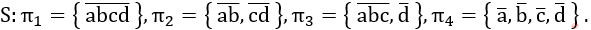Let p be the partial order on the set of partitions S' = {π1, π2, π3, π4} defined as follows: πi p πj if and only if πi refines πj. The poset diagram for (S', p) is:

 AB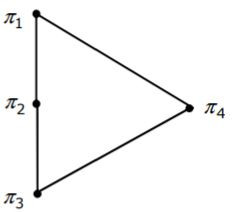CDEngineering-Mathematics       Sets-And Relation
Question 26 Explanation:
π4 = refines every partition. So it has to be bottom of poset diagram.
And, neither π2 refines π3, nor π3 refines π2.
Here, only π1 refined by every set, so it has to be at the top.
Finally, option C satisfies all the property.
 Question 27

Consider the set of (column) vectors defined by X = {x ∈ R3| x1+x2+x3 = 0, where xT = [x1,x2,x3]T}. Which of the following is TRUE?

 A {[1,-1,0]T, [1,0,-1]T} is a basis for the subspace X. B {[1,-1,0]T, [1,0,-1]T} is a linearly independent set, but it does not span X and therefore is not a basis of X. C X is not a subspace of R3 D None of the above
Engineering-Mathematics       Linear-Algebra
Question 27 Explanation:
Since, [1,-1,0]T and [1,0,-1]T are linearly independent set and spans X. So is the basis for subspace X.
 Question 28

Consider the series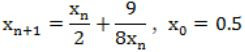obtained from the Newton-Raphson method. The series converges to

 A 1.5 B √2 C 1.6 D 1.4
Engineering-Mathematics       Newton-Raphson-Method
Question 28 Explanation:
Given series is
xn+1 = xn/2+9/8xn ⟶ (I); x0 = 0.5
Equation based on Newton-Raphson is
xn+1 = xn-f(xn)/f'(xn) ⟶ (II)
Equate I and II
xn-f(xn)/f'(xn) = xn/2+9/8xn
xn-f(xn)/f'(xn) = xn-xn/2+9/8xn
xn-f(xn)/f'(xn) = xn-(4xn2-9)/8xn
So, f(x) = 4xn2-9
4x2-9 = 0
4x2 = 9
x2 = 9/4
x = ±3/2
x=±1.5
 Question 29

A minimum state deterministic finite automaton accepting the language L = {w | w ε {0,1} *, number of 0s and 1s in w are divisible by 3 and 5, respectively} has

 A 15 states B 11 states C 10 states D 9 states
Theory-of-Computation       Finite-Automata
Question 29 Explanation:
Given that number of 0’s and 1’s are divisible by 3 and 5, it means that the number of 0’s and 1’s must be divisible by 15. As the LCM of 3 and 5 is 15, so number of 0’s and 1’s are divisible by 3 and 5 is only possible if of 0’s and 1’s are divisible by 15. Also modulo 3 will leave a remainder of 0,1,2 (3 states required) and modulo 5 will leave remainder of 0,1,2,3,4 (5 states required), so product automata will require (3 × 5=15 states).
 Question 30

The language L = {0i21i | i≥0} over the alphabet {0, 1, 2} is:

 A not recursive. B is recursive and is a deterministic CFL. C is a regular language. D is not a deterministic CFL but a CFL.
Theory-of-Computation       Identify-Class-Language
Question 30 Explanation:
We have to match number 0’s before 2 and number of 1’s after 2, both must be equal in order to string belongs to language. This can be done by deterministic PDA. First we have to push 0’s in stack, when “2” comes , ignore it and after for each 1’s we have to pop one “0” from stack. If stack and input string both are empty at the same time then the string will be accepted else rejected. NOTE: i>=0 , so a single “2” is also accepted by DPDA. Hence this language is DCFL and every DCFL is recursive also, so it is also a recursive language.
 Question 31

Which of the following languages is regular?

 A {wwR|w ∈ {0,1}+} B {wwRx|x, w ∈ {0,1}+} C {wxwR|x, w ∈ {0,1}+} D {xwwR|x, w ∈ {0,1}+}
Theory-of-Computation       Regular-Language
Question 31 Explanation:
The regular expression corresponding to option C is:
0 (0+1)+ 0 + 1 (0+1)+ 1
Any string which begins and ends with same symbol, can be written in form of “wxwr
For example consider a string: 10010111, in this string “w=1” , “x= 001011” and wr = 1.
Hence any string which begins and ends with either “0” or with “1” can be written in form of “wxwr” and L = {wxwr | x,w ϵ {0,1}+} is a regular language.
 Question 32

Let f(w, x, y, z) = ∑(0, 4, 5, 7, 8, 9, 13, 15). Which of the following expressions are NOT equivalent to f?

(P) x'y'z' + w'xy' + wy'z + xz
(Q) w'y'z' + wx'y' + xz
(R) w'y'z' + wx'y' + xyz + xy'z
(S) x'y'z' + wx'y' + w'y
 A P only B Q and S C R and S D S only
Digital-Logic-Design       Boolean-Expressions
Question 32 Explanation:(P), (Q), (R) cover all the minterms and are equivalent to f(w,x,y,z) = Σ(0,4,5,7,8,9,13,15).
(S) covers the minterms m0, m8, m9, m2, m3, m6, m7.
(S) is not covering the minterms m4, m5, m13, m15.
 Question 33

Define the connective * for the Boolean variables X and Y as: X * Y = XY + X'Y'. Let Z = X * Y.

Consider the following expressions P, Q and R.

```P: X = Y⋆Z
Q: Y = X⋆Z
R: X⋆Y⋆Z = 1```

Which of the following is TRUE?

 A Only P and Q are valid. B Only Q and R are valid. C Only P and R are valid. D All P, Q, R are valid.
Digital-Logic-Design       Boolean-Expressions
Question 33 Explanation:
P: Y*Z = YZ + Y’Z’
= Y(XY + X’Y’) + Y’(XY+X’Y’)’
= XY+Y’(X ⊕ Y)
= XY+Y’(XY’+X’Y)
= XY+XY’
= X(Y+Y’) = X
Q: X*Z = (XZ + X’Z’)
= X(XY + X’Y’) + X’(XY + X’Y’)’
= XY+X’(X’Y+XY’)
= XY+X’Y
= (X+X’)Y = Y
R: X* Y*Z
= X*X Since P: Y*Z= X
= XX + X’X’
= 1
 Question 34

Suppose only one multiplexer and one inverter are allowed to be used to implement any Boolean function of n variables. What is the minimum size of the multiplexer needed?

 A 2n line to 1 line B 2n+1 line to 1 line C 2n-1 line to 1 line D 2n-2 line to 1 line
Digital-Logic-Design       Multiplexer
Question 34 Explanation:
Both true and complement forms of all variables are necessary to implement any function of n variables.
A 2n X 1 multiplexer can implement any function of n variables. As n variables are given to select lines, so that true and complement forms of all variables get generated inside the MUX.
As one inverter is available, we can generate complement of one variable outside of the Multiplexer. And remaining (n-1) variables are given to select lines. With this we have true and complement form of all n variables.
So, the answer is 2n-1 X 1 MUX.
 Question 35

In a look-ahead carry generator, the carry generate function Gi and the carry propagate function Pi for inputs Ai and Bi are given by:

`Pi = Ai ⨁ Bi and Gi = AiBi`

The expressions for the sum bit Si and the carry bit Ci+1 of the look-ahead carry adder are given by:

`Si = Pi ⨁ Ci and Ci+1 = Gi + PiCi , where C0 is the input carry.`

Consider a two-level logic implementation of the look-ahead carry generator. Assume that all Pi and Gi are available for the carry generator circuit and that the AND and OR gates can have any number of inputs. The number of AND gates and OR gates needed to implement the look-ahead carry generator for a 4-bit adder with S3, S2, S1, S0 and C4 as its outputs are respectively:

 A 6, 3 B 10, 4 C 6, 4 D 10, 5
Digital-Logic-Design       Carry-Look-Ahead-Adder
Question 35 Explanation:
Formula: n(n+1)/2 AND gates and n OR gates are needed for an n-bit carry look ahead circuit for addition of two binary numbers.
 Question 36

The control signal functions of a 4-bit binary counter are given below (where X is “don’t care”) The counter is connected as follows: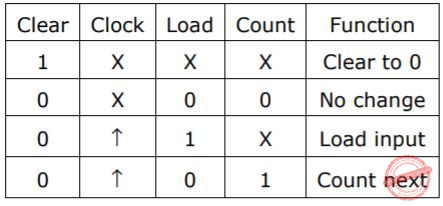The counter is connected as follows:Assume that the counter and gate delays are negligible. If the counter starts at 0, then it cycles through the following sequence:

 A 0, 3, 4 B 0, 3, 4, 5 C 0, 1, 2, 3, 4 D 0, 1, 2, 3, 4, 5
Digital-Logic-Design       Circuits-Output
Question 36 Explanation:
A counter goes through a sequence of states. Given counter resets when A1 = A3 = 1.
Here, initial state is 0000. It goes through 0001,0010,0011,0100 and 0101. When the state is 5(0101) it immediately resets to initial state 0. Here, state 5 is not considered as valid state.
So valid states are 0,1,2,3, and 4 and hence it is a Mod5 counter.
 Question 37

Consider a pipelined processor with the following four stages:

```  IF: Instruction Fetch
ID: Instruction Decode and Operand Fetch
EX: Execute
WB: Write Back```

The IF, ID and WB stages take one clock cycle each to complete the operation. The number of clock cycles for the EX stage depends on the instruction. The ADD and SUB instructions need 1 clock cycle and the MUL instruction needs 3 clock cycles in the EX stage. Operand forwarding is used in the pipelined processor. What is the number of clock cycles taken to complete the following sequence of instructions?

```  ADD R2, R1, R0       R2 <- R0 + R1
MUL R4, R3, R2       R4 <- R3 * R2
SUB R6, R5, R4       R6 <- R5 - R4```
 A 7 B 8 C 10 D 14
Computer-Organization       Pipelining
Question 37 Explanation:
Since operand forwarding is there, by default we consider the operand forwarding from EX stage to EX stage.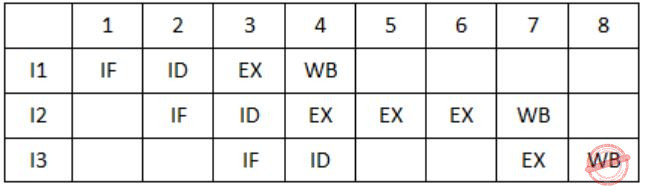So, total no. of clock cycles needed to execute the given 3 instructions is 8.
 Question 38

The following postfix expression with single digit operands is evaluated using a stack:

`            8 2 3 ^ / 2 3 * + 5 1 * -  `

Note that ^ is the exponentiation operator. The top two elements of the stack after the first * is evaluated are:

 A 6, 1 B 5, 7 C 3, 2 D 1, 5
Data-Structures       Stacks
Question 38 Explanation:
8 2 3 ^ / 2 3 * + 5 1 * -After the * is evaluated at the time elements in the stack is 1, 6.
 Question 39

The inorder and preorder traversal of a binary tree are d b e a f c g and a b d e c f g, respectively. The postorder traversal of the binary tree is:

 A d e b f g c a B e d b g f c a C e d b f g c a D d e f g b c a
Data-Structures       Binary-Trees
Question 39 Explanation:
Inorder: Left root Right
Pre order: Root Left Right
Post order: Left Right Root
Inorder: d b e a f c g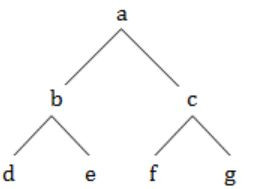Pre order: a b d e c f g
Post order: d e b f g c a
 Question 40

Consider a hash table of size seven, with starting index zero, and a hash function (3x + 4) mod 7. Assuming the hash table is initially empty, which of the following is the contents of the table when the sequence 1, 3, 8, 10 is inserted into the table using closed hashing? Note that ‘_’ denotes an empty location in the table.

 A 8, _, _, _, _, _, 10 B 1, 8, 10, _, _, _, 3 C 1, _, _, _, _, _,3 D 1, 10, 8, _, _, _, 3
Data-Structures       Hashing
Question 40 Explanation:
Consider a hash table of size 7
Starting index is zero i.e.,⇾ Given hash function is = (3x+4) mod 3
⇾ Given sequence is = 1, 3, 8, 10
where x = 1 ⟹ (3(1)+4)mod 3 = 0
1 will occupy index 0.
where x = 3 ⟹ (3(3)+4) mod 7 = 6
3 will occupy index 6.
where x = 8 ⟹ (3(8)+4) mod 7 = 0
Index ‘0’ is already occupied then put value(8) at next space (1).
where x = 10 ⟹ (3(10)+4) mod 7 = 6
Index ‘6’ is already occupied then put value(10) at next space (2).
The resultant sequence is (1, 8, 10, __ , __ , __ , 3).
 Question 41

In an unweighted, undirected connected graph, the shortest path from a node S to every other node is computed most efficiently, in terms of time complexity, by

 A Dijkstra’s algorithm starting from S. B Warshall’s algorithm. C Performing a DFS starting from S. D Performing a BFS starting from S.
Algorithms        Time-Complexity
Question 41 Explanation:
→ Time Complexity of the Dijkstra’s algorithm : It depends on your implementation of Dijkstra's Algorithm. Simple algorithm is given below with Time complexity of O(V2). There are also some time-efficient Algorithms: Graph represented using adjacency list can be reduced to O(E log V) with the help of binary heap.
→ Time Complexity of the Warshall’s algorithm: O(n3). Warshall’s algorithm basically we are using to find all pair shortest path.
→ DFS cannot be used for finding shortest paths.
→ Time Complexity for BFS : O(E+V)
 Question 42

Consider the following C function:

#include
```int f(int n)
{
static int r = 0;
if (n <= 0) return 1;
if (n > 3)
{
r = n;
return f(n-2)+2;
}
return f(n-1)+r;
}

int main()
{
printf("%d", f(5));
}```

What is the value of f(5)?

 A 5 B 7 C 9 D 18
Programming       C-Programming
Question 42 Explanation:
We need to evaluate f(5)
f(5) = f(3) + 2
f(3) = f(2) + 5 (where r is static and value of r=5)
f(2) = f(1) + 5
f(1) = f(0) + 5
f(0) = 1
⟹ f(5) = 1+5+5+5+2 = 18
 Question 43

A complete n-ary tree is a tree in which each node has n children or no children. Let I be the number of internal nodes and L be the number of leaves in a complete n-ary tree. If L = 41, and I = 10, what is the value of n?

 A 3 B 4 C 5 D 6
Data-Structures       N-array-Tree
Question 43 Explanation:
L = (n-1) * I + 1
L = No. of leaves = 41
I = No. of Internal nodes = 10
41 = (n-1) * 10 + 1
40 = (n-1) * 10
n = 5
 Question 44

In the following C function, let n >= m.

```int gcd(n,m)
{
if (n%m ==0) return m;
n = n%m;
return gcd(m,n);
} ```

How many recursive calls are made by this function?

 A θ (log2 n) B Ω (n) C θ (log2log2 n) D θ(√n)
Algorithms        Time-Complexity
Question 44 Explanation:
The given code is to calculate the greatest common divisor (GCD) using Euclidean algorithm.
Then, time complexity is = O(log(a∙b) = O(log(a+b)) = O(log n)
 Question 45

What is the time complexity of the following recursive function:

```int DoSomething (int n) {
if (n <= 2)
return 1;
else
return (DoSomething (floor(sqrt(n))) + n);
}```
 A Ω (n2) B θ (nlog2 n) C θ (log2 n) D θ (log2log2 n)
Algorithms        Time-Complexity
Question 45 Explanation:Now apply Master's theorem,
a=1, b=2, k=0, p=0
a = bk, so Case-2 will be applied, and p>-1, soQuestion 46

Consider the following C program segment where CellNode represents a node in a binary tree:

```  struct CellNode {
struct CellNOde *leftChild;
int element;
struct CellNode *rightChild;
};

int GetValue(struct CellNode *ptr) {
int value = 0;
if (ptr != NULL)            {
if ((ptr->leftChild == NULL) &&
(ptr->rightChild == NULL))
value = 1;
else
value = value + GetValue(ptr->leftChild)
+ GetValue(ptr->rightChild);
}
return(value);
}
The value returned by GetValue() when a pointer to the root of a binary tree is passed as its argument is:```
 A the number of nodes in the tree B the number of internal nodes in the tree C the number of leaf nodes in the tree D the height of the tree
Data-Structures       Binary-Trees
Question 46 Explanation:
Let take example,So from applying algorithm to above tree we got the final value v=3 which is nothing but no. of leaf nodes.
Note that height of the tree is also 3 but it is not correct because in algorithm the part
if ((ptr → leafchild = = NULL) && (ptr → rightchild = = NULL)) value = 1;
Says that if there is only one node the value is 1 which cannot be height of the tree because the tree with one node has height '0'.
 Question 47

Consider the process of inserting an element into a Max Heap, where the Max Heap is represented by an array. Suppose we perform a binary search on the path from the new leaf to the root to find the position for the newly inserted element, the number of comparisons performed is:

 A θ(log2n) B θ(log2log2n) C θ(n) D θ(nlog2n)
Data-Structures       Heap-Tree
Question 47 Explanation:
Max heap is the complete binary tree that means each node has either zero children or two children except last level. So in worst case insertion of element is at last level. So, number of comparisons required at each level starting from root is equal to 1+2+4+8+16+---- this series is equal to "logn". All the elements are sorted, the binary search which will result in O(loglogn) number of comparisons.
 Question 48

Which of the following is TRUE about formulae in Conjunctive Normal Form?

 A For any formula, there is a truth assignment for which at least half the clauses evaluate to true. B For any formula, there is a truth assignment for which all the clauses evaluate to true. C There is a formula such that for each truth assignment, at most one-fourth of the clauses evaluate to true. D None of the above.
Digital-Logic-Design       Conjunctive-Normal-Form
Question 48 Explanation:
For n=2, (means two variables a and b)
Formula: a ∧ b
Truth table:Conjunctive normal form : (a ∨ b) ∧ (a ∨ ~b) ∧ (~a ∨ b)Similarly,
For n=1-----TRUE=1, FALSE=1 (1/2 ARE TRUE)
For n=2-----TRUE=3, FALSE=1 (3/4 ARE TRUE)
For n=3-----TRUE=7, FALSE=1 (7/8 ARE TRUE)
(1-2-n) are TRUE.
Looking at options,Question 49

Let w be the minimum weight among all edge weights in an undirected connected graph. Let e be a specific edge of weight w. Which of the following is FALSE?

 A There is a minimum spanning tree containing e. B If e is not in a minimum spanning tree T, then in the cycle formed by adding e to T, all edges have the same weight. C Every minimum spanning tree has an edge of weight w. D e is present in every minimum spanning tree.
Algorithms        Minimum-Spanning-Tree
Question 49 Explanation:
To find minimum Spanning tree(MST), may not be present ‘e’ in every MST graph.
 Question 50

An array of n numbers is given, where n is an even number. The maximum as well as the minimum of these n numbers needs to be determined. Which of the following is TRUE about the number of comparisons needed?

 A At least 2n - c comparisons, for some constant c, are needed. B At most 1.5n - 2 comparisons are needed. C At least nlog2n comparisons are needed. D None of the above.
Algorithms        Time-Complexity
Question 50 Explanation:
→ Take first two elements of an array and compare them and mark one of them as max and min for another one. So for now one comparision required.
→ Now take other two element and compare between them and we will get one minimum element which will be compared with min and we will get one maximum element which will be compared with max and accordingly we will mark them. So in total 3 comparisions are required, and we will do this with all (n-1)/2 pairs.
So in total, no. of comparisions required,
= 3(n-2)/2 + 2
= 3n/2 - 3 + 2
= 3n/2 - 1
= 1.5n - 1
 Question 51

Consider the following C code segment:

```   int IsPrime(n)
{
int i,n;
for(i=2;i<=sqrt(n);i++)
if(n%i == 0)
{printf(“Not Prime\n”); return 0;}
return 1;
}```

Let T(n) denotes the number of times the for loop is executed by the program on input n. Which of the following is TRUE?>/p>

 A T(n) = O(√n) and T(n) = Ω(√n) B T(n) = O(√n) and T(n) = Ω(1) C T(n) = O(n) and T(n) = Ω(√n) D None of the above
Algorithms        Time-Complexity
Question 51 Explanation:
Here in best case time complexity is omega(1) and in worst case time complexity is O(sqrt(n)).
 Question 52

Consider the grammar with non-terminals N = {S,C,S1},terminals T = {a,b,i,t,e}, with S as the start symbol, and the following set of rules:

```      S --> iCtSS1|a
S1 --> eS|ϵ
C --> b```

The grammar is NOT LL(1) because:

 A it is left recursive B it is right recursive C it is ambiguous D it is not context-free
Compiler-Design       Parsers
Question 52 Explanation:
The given grammar is not left recursive and also it is context free (Type 2 grammar), so option A and D is wrong. Being a right recursive grammar is not an issue for LL(1) grammar. So even if given grammar is right recursive, this is not a reason for NOT LL(1).
This grammar has two parse tree for string “ibt ibt aea”.Question 53

Consider the following two statements:

```P: Every regular grammar is LL(1)
Q: Every regular set has a LR(1) grammar```

Which of the following is TRUE?

 A Both P and Q are true B P is true and Q is false C P is false and Q is true D Both P and Q are false
Compiler-Design       Parsers
Question 53 Explanation:
Every regular grammar is LL(1) is false, as the grammar may have left recursion or left factoring or also it is possible that grammar is ambiguous.
For ex: Consider a regular grammar
S -> aS | a | ϵ
this grammar is ambiguous as for string "a" two parse tree is possible.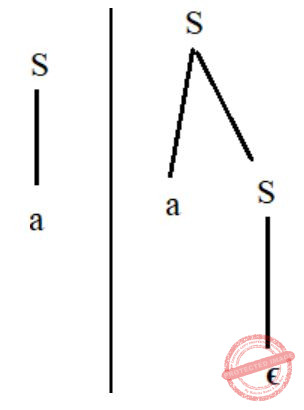Hence it is regular but not LL(1).
But every regular set has a language accept or as DFA , so every regular set must have atleast one grammar which is unambiguous.
Hence, every regular set has LR(1) grammar.
 Question 54

In a simplified computer the instructions are:

```  OP Rj,Ri - Performs Rj OP Ri and stores the result in register Ri.
OP m,Ri  - Performs val OP Ri and stores the result in Ri. val denotes the content of memory location m.
MOV m,Ri - Moves the content of memory location m to register Ri.
MOV Ri,m - Moves the content of register Ri to memory location m.```

The computer has only to registers, and OP is either ADD or SUB. Consider the following basic block:

```  t1 = a+b
t2 = c+d
t3 = e-t2
t4 = t1-t3 ```

Assume that all operands are initially in memory. The final value of the computation should be in memory. What is the minimum number of MOV instructions in the code generated for this basic block?

 A 2 B 3 C 5 D 6
Computer-Organization       Machine-Instructions
Question 54 Explanation:
We can write the given four instructions using OP and MOV operations as below.
MOV a, R1
ADD b, R1
MOV c, R2
ADD d, R2
SUB e, R2
SUB R1, R2
MOV R2, m
So, from the above total no. of MOV instructions = 3
 Question 55

An operating system uses Shortest Remaining Time first (SRT) process scheduling algorithm. Consider the arrival times and execution times for the following processes:

```
Process     Execution time     Arrival time
P1             20                0
P2             25                15
P3             10                30
P4             15                45
```

What is the total waiting time for process P2?

 A 5 B 15 C 40 D 55
Operating-Systems       Process-Scheduling
Question 55 Explanation:Waiting time for process P2 = TAT - Execution time
= Completion time - AT - ET
= 55 - 15 - 25
= 15
 Question 56

A virtual memory system uses First In First Out (FIFO) page replacement policy and allocates a fixed number of frames to a process. Consider the following statements:

```P: Increasing the number of page frames allocated to a
process sometimes increases the page fault rate.
Q: Some programs do not exhibit locality of reference.```

Which one of the following is TRUE?

 A Both P and Q are true, and Q is the reason for P B Both P and Q are true, but Q is not the reason for P C P is false, but Q is true D Both P and Q are false
Operating-Systems       Page-Replacement
Question 56 Explanation:
Statement P,
FIFO suffers from Belady anomaly. Belady anomaly states that when number of page frames increases no. of page fault increases.
Statement Q,
Locality of reference also known as the principle of locality, is the tendency of a processor to accesses the same set of memory locations respectively over a short period of time.
And yes some programs do not exhibit locality of reference.
Hence, both P and Q are true. But Q is not the reason for P.
 Question 57

A single processor system has three resource types X, Y and Z, which are shared by three processes. There are 5 units of each resource type. Consider the following scenario, where the column alloc denotes the number of units of each resource type allocated to each process, and the column request denotes the number of units of each resource type requested by a process in order to complete execution. Which of these processes will finish LAST?

```
alloc      request
X Y Z       X Y Z
P0  1 2 1       1 0 3
P1  2 0 1       0 1 2
P2  2 2 1       1 2 0```
 A P0 B P1 C P2 D None of the above, since the system is in a deadlock.
Operating-Systems       Bankers-Algorithm
Question 57 Explanation:
Given that there are 5 units of each resource type.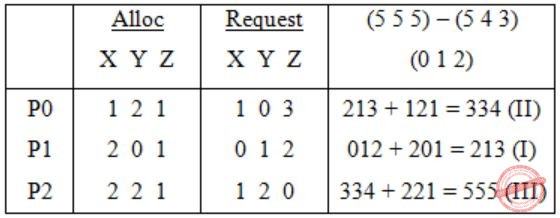543:
System is in safe state
Order of Execution =
P2 will execute last.
 Question 58

Two processes, P1 and P2, need to access a critical section of code. Consider the following synchronization construct used by the processes:

```  /* P1 */
while (true) {
wants1 = true;
while (wants2 == true);
/* Critical
Section */
wants1=false;
}
/* Remainder section */

/* P2 */
while (true) {
wants2 = true;
while (wants1==true);
/* Critical
Section */
wants2 = false;
}
/* Remainder section */ ```

Here, wants1 and wants2 are shared variables, which are initialized to false. Which one of the following statements is TRUE about the above construct?

 A It does not ensure mutual exclusion. B It does not ensure bounded waiting. C It requires that processes enter the critical section in strict alternation. D It does not prevent deadlocks, but ensures mutual exclusion.
Operating-Systems       Process-Synchronization
Question 58 Explanation:
First of all it can be easily seen that mutual exclusion is satisfied.
Now, if in P1
wants1 = true ;
executed and preempted and now if in P2
wants2 = true ;
executed.
Then the deadlock situation will be created because both will fall into infinite loop.
 Question 59

Information about a collection of students is given by the relation studinfo(studId, name, sex). The relation enroll(studId, courseId) gives which student has enrolled for (or taken) that course(s). Assume that every course is taken by at least one male and at least one female student. What does the following relational algebra expression represent?

ΠcourseId((ΠstudIdsex="female"(studInfo))×ΠcourseId(enroll))-enroll)
 A Courses in which all the female students are enrolled. B Courses in which a proper subset of female students are enrolled. C Courses in which only male students are enrolled. D None of the above
Database-Management-System       Relational-Algebra
Question 59 Explanation:
Option A: It is not result the all the female students who are enrolled. So option A is false.
Option B: Yes, True. It selects the proper subset of female students which are enrolled because in the expression we are performing the Cartesian product.
Option C: False. It doesn’t shows (or) display the males students who are enrolled.
 Question 60

Consider the relation employee(name, sex, supervisorName) with name as the key. supervisorName gives the name of the supervisor of the employee under consideration. What does the following Tuple Relational Calculus query produce?

```{e.name|employee(e)∧}
(∀x)[¬employee(x) ∨ x.supervisorName ≠ e.name ∨ x.sex = "male"]}```
 A Names of employees with a male supervisor. B Names of employees with no immediate male subordinates. C Names of employees with no immediate female subordinates. D Names of employees with a female supervisor.
Database-Management-System       Relational-Calculus
Question 60 Explanation:
The given Tuple Relational calculus produce names of employees with no immediate female subordinates.
 Question 61

Consider the table employee(empId, name, department, salary) and the two queries Q1,Q2 below. Assuming that department 5 has more than one employee, and we want to find the employees who get higher salary than anyone in the department 5, which one of the statements is TRUE for any arbitrary employee table?

```Q1 : Select e.empId
From employee e
Where not exists
(Select * From employee s where s.department = “5” and s.salary >= e.salary)
Q2 : Select e.empId
From employee e
Where e.salary > Any
(Select distinct salary From employee s Where s.department = “5”)```
 A Q1 is the correct query B Q2 is the correct query C Both Q1 and Q2 produce the same answer D Neither Q1 nor Q2 is the correct query
Question 61 Explanation:
The required output is find the employees who get higher salary than anyone in the department “5”.
Query 1: Results the empId's which have higher salary than anyone in the department 5.
Query 2: Results the empId's which have higher salary than atleast one employee of department 5.
 Question 62

Which one of the following statements if FALSE?

 A Any relation with two attributes is in BCNF B A relation in which every key has only one attribute is in 2NF C A prime attribute can be transitively dependent on a key in a 3NF relation D A prime attribute can be transitively dependent on a key in a BCNF relation
Database-Management-System       Normalization
Question 62 Explanation:
Rules for BCNF is:
i) It is in 3NF.
ii) For any dependency X→ Y
where X is a super key.
iii) Functional dependency has been removed.
Option D is false.
→ Because a prime attribute can’t be transitive dependent on a key in a BCNF relation.
 Question 63

The order of a leaf node in a B+- tree is the maximum number of (value, data record pointer) pairs it can hold. Given that the block size is 1K bytes, data record pointer is 7 bytes long, the value field is 9 bytes long and a block pointer is 6 bytes long, what is the order of the leaf node?

 A 63 B 64 C 67 D 68
Database-Management-System       B+-Trees
Question 63 Explanation:
Disk Block size = 1 KBytes = 210 Bytes = 1024 Bytes
Data recorder pointer size, r = 7 bytes
Value size, v = 9 bytes
Disk Block pointer, P = 6 bytes
⇒ Order of leaf be m, then
r*m+v*m+p <= 1024
7m+9m+6 <= 1024
16m <= 1024 - 6
m <= 63
 Question 64

Consider the following schedules involving two transactions. Which one of the following statements is TRUE?

S1: r1(X); r1(Y); r2(X); r2(Y); w2(Y); w1(X)
S2: r1(X); r2(X); r2(Y); w2(Y); r1(Y); w1(X)
 A Both S1 and S2 are conflict serializable. B S1 is conflict serializable and S2 is not conflict serializable. C S1 is not conflict serializable and S2 is conflict serializable. D Both S1 and S2 are not conflict serializable.
Database-Management-System       Transactions
Question 64 Explanation:In precedence graph of S1 since cycle is formed so not conflict serializable.
But in precedence graph of S2 No cycle is formed so it is conflict serializable.
 Question 65

There are n stations in a slotted LAN. Each station attempts to transmit with a probability p in each time slot. What is the probability that ONLY one station transmits in a given time slot?

 A np(1-p)n-1 B (1-p)n-1 C p(1-p)n-1 D 1-(1-p)n-1
Computer-Networks       LAN-and-Probability
Question 65 Explanation:
This question can be solved by binomial distribution,
nC1P1(1-p)n-1
= nP(1-P)n-1
 Question 66

In a token ring network the transmission speed is 107 bps and the propagation speed is 200 metres/μs. The 1-bit delay in this network is equivalent to:

 A 500 metres of cable. B 200 metres of cable. C 20 metres of cable. D 50 metres of cable.
Computer-Networks       Token-Ring-Network
Question 66 Explanation:
Tt = L / B => 1/ 107 = 0.1 microsec
Given Tp = 200 m / microsec
In, 1 microsec it covers 200m
Therefore, in 0.1 microsec it is 200 * 0.1 = 20 meters
 Question 67

The address of a class B host is to be split into subnets with a 6-bit subnet number. What is the maximum number of subnets and the maximum number of hosts in each subnet?

 A 62 subnets and 262142 hosts. B 64 subnets and 262142 hosts. C 62 subnets and 1022 hosts. D 64 subnets and 1024 hosts.
Computer-Networks       IP-Address
Question 67 Explanation:
It is a class B address, so there 16-bits for NID and 16-bits for HID.
From HID, we took 6-bits for subnetting.
Then total subnets possible = ( 26 ) - 2 = 64
Total hosts possible for each subnet = (210) - 2 = 1022
 Question 68

The message 11001001 is to be transmitted using the CRC polynomial x3+1 to protect it from errors. The message that should be transmitted is:

 A 11001001000 B 11001001011 C 11001010 D 110010010011
Computer-Networks       CRC
Question 68 Explanation:
CRC polynomial = x3+1 [∵ In data 3-zero’s need to be append to data]
= 1001∴ Data transmitted is: 11001001011
 Question 69

The distance between two stations M and N is L kilometers. All frames are K bits long. The propagation delay per kilometer is t seconds. Let R bits/second be the channel capacity. Assuming that processing delay is negligible, the minimum number of bits for the sequence number field in a frame for maximum utilization, when the sliding window protocol is used, is:

 A ⌈log2(2LtR+2K/K)⌉ B ⌈log2(2LtR/K)⌉ C ⌈log2(2LtR+K/K)⌉ D ⌈log2(2LtR+K/2K)⌉
Computer-Networks       Sliding-Window-Protocol
Question 69 Explanation:
Maximum window size at sender side,
N = (Tk + 2Tp)/Tk
Tp = l × l sec
Tk = K/R sec
So, N = (K/R+2Lt)/(K/R) = K+2LtR/K
Note that when asked for general sliding window protocol (not GBN nor SR) then we do not care about receiver's window size.
So, no. of bits required,
⌈log2 K+2LtR/K⌉
 Question 70

Match the following:

```     (P) SMTP     (1) Application layer
(Q) BGP      (2) Transport layer
(R) TCP      (3) Data link layer
(S) PPP      (4) Network layer
(5) Physical layer```
 A P – 2 Q – 1 R – 3 S – 5 B P – 1 Q – 4 R – 2 S – 3 C P – 1 Q – 4 R – 2 S – 5 D P – 2 Q – 4 R – 1 S – 3
Computer-Networks       Match-the-Following
Question 70 Explanation:
P) SMTP is used for email transmission.
Q) BGP is network layer protocol that manages low packets are routed across the network.
R) TCP is a transport layer protocol.
S) PPP is a data link layer protocol.
 Question 71

Consider the following program segment. Here R1, R2 and R3 are the general purpose registers.

```       Instruction      Operation     Instruction size (no. of words)
MOV R1,(3000)   R1 ← m                2
LOOP: MOV R2,(R3)     R2 ← M[R3]                  1
ADD R2,R1       R2 ← R1 + R2                1
MOV (R3),R2     M[R3] ← R2                  1
INC R3          R3 ← R3 + 1                 1
DEC R1          R1 ← R1 - 1                 1
BNZ LOOP        Branch on not zero          2
HALT            Stop                        1 ```

Assume that the content of memory location 3000 is 10 and the content of the register R3 is 2000. The content of each of the memory locations from 2000 to 2010 is 100. The program is loaded from the memory location 1000. All the numbers are in decimal.

Assume that the memory is word addressable. The number of memory references for accessing the data in executing the program completely is:

 A 10 B 11 C 20 D 21
Computer-Organization       Machine-Instructions
Question 71 Explanation:
Firstly before the loop one memory reference is,
R1 ← m
Now loop will run 10 times because R1 contain value 10, and in each iteration of the loop there are two memory reference,
R2 ← M[R3]
M[R3] ← R2
So, in total, the no. of memory references are,
2(10) + 1 = 21
 Question 72

Consider the following program segment. Here R1, R2 and R3 are the general purpose registers.

```       Instruction      Operation     Instruction size (no. of words)
MOV R1,(3000)   R1 ← m                2
LOOP: MOV R2,(R3)     R2 ← M[R3]                  1
ADD R2,R1       R2 ← R1 + R2                1
MOV (R3),R2     M[R3] ← R2                  1
INC R3          R3 ← R3 + 1                 1
DEC R1          R1 ← R1 - 1                 1
BNZ LOOP        Branch on not zero          2
HALT            Stop                        1 ```

Assume that the content of memory location 3000 is 10 and the content of the register R3 is 2000. The content of each of the memory locations from 2000 to 2010 is 100. The program is loaded from the memory location 1000. All the numbers are in decimal.

Assume that the memory is word addressable. After the execution of this program, the content of memory location 2010 is:

 A 100 B 101 C 102 D 110
Computer-Organization       Machine-Instructions
Question 72 Explanation:
Loop will execute 10 times since the value in R1 stored is 10 in first step.
Now since the value will change at memory locations from 2000 to 2009 and will not affect the memory locations 2010.
Hence, the value at memory location 2010 it will be old value, i.e., 100.
 Question 73

Consider the following program segment. Here R1, R2 and R3 are the general purpose registers.

```       Instruction      Operation     Instruction size (no. of words)
MOV R1,(3000)   R1 ← m                2
LOOP: MOV R2,(R3)     R2 ← M[R3]                  1
ADD R2,R1       R2 ← R1 + R2                1
MOV (R3),R2     M[R3] ← R2                  1
INC R3          R3 ← R3 + 1                 1
DEC R1          R1 ← R1 - 1                 1
BNZ LOOP        Branch on not zero          2
HALT            Stop                        1 ```

Assume that the content of memory location 3000 is 10 and the content of the register R3 is 2000. The content of each of the memory locations from 2000 to 2010 is 100. The program is loaded from the memory location 1000. All the numbers are in decimal.

Assume that the memory is byte addressable and the word size is 32 bits. If an interrupt occurs during the execution of the instruction “INC R3”, what return address will be pushed on to the stack?

 A 1005 B 1020 C 1024 D 1040
Computer-Organization       Machine-Instructions
Question 73 Explanation:
Memory is byte addressable, so it requires 1 word or 4 bytes to perform a each operation such that
→ Starts at memory location 1000.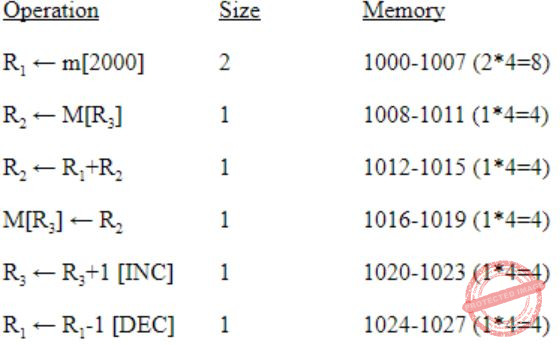Interrupt occurs during the execution of information “INC R3”.
Then the value of address i.e., 1024 is pushed into the stack.
 Question 74

Consider the following Finite State Automaton.The language accepted by this automaton is given by the regular expression

 A b*ab*ab*ab* B (a+b)* C b*a(a+b)* D b*ab*ab*
Theory-of-Computation       Finite-Automata
Question 74 Explanation:
State q3 is unreachable and state q1 and q2 are equivalent, so we can merge q1 and q2 as one state. The resulting DFA will be: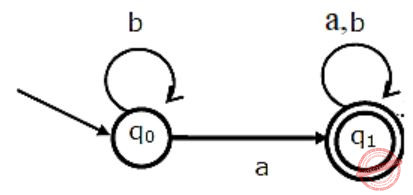Clearly we can see that the regular expression for DFA is “ b*a (a+b)* ”.
 Question 75

Consider the following Finite State Automaton.The minimum state automaton equivalent to the above FSA has the following number of states

 A 1 B 2 C 3 D 4
Theory-of-Computation       Finite-Automata
Question 75 Explanation:
The minimum state automaton for problem 74 is:Question 76

Suppose the letters a, b, c, d, e, f have probabilities 1/2, 1/4, 1/8, 1/16, 1/32, 1/32 respectively.

Which of the following is the Huffman code for the letter a, b, c, d, e, f?

 A 0, 10, 110, 1110, 11110, 11111 B 11, 10, 011, 010, 001, 000 C 11, 10, 01, 001, 0001, 0000 D 110, 100, 010, 000, 001, 111
Algorithms        Huffman-Coding
Question 76 Explanation:→ 0, 10, 110, 1110, 11110, 11111
 Question 77

Suppose the letters a, b, c, d, e, f have probabilities 1/2, 1/4, 1/8, 1/16, 1/32, 1/32 respectively.

What is the average length of Huffman codes?

 A 3 B 2.1875 C 2.25 D 1.9375
Algorithms        Huffman-Coding
Question 77 Explanation:
The Average length =(1*1/2+2*1/4+3*1/8+4*1/16+5*1/32+5*1/32) = 1.9375
 Question 78

Consider the CFG with {S,A,B} as the non-terminal alphabet, {a,b} as the terminal alphabet, S as the start symbol and the following set of production rules:

```S → aB        S → bA
B → b         A → a
B → bS        A → aS
B → aBB       A → bAA```

Which of the following strings is generated by the grammar?

 A aaaabb B aabbbb C aabbab D abbbba
Compiler-Design       Grammar
Question 78 Explanation:
The string “aabbab” can be derived by following steps:
S -> aB [Using S --> aB]
-> aaBB [Using B --> aBB]
-> aabB [Using B --> b]
-> aabbS [Using B --> bS]
-> aabbaB [Using S --> aB]
-> aabbab [Using B --> b]
 Question 79

Consider the CFG with {S,A,B} as the non-terminal alphabet, {a,b} as the terminal alphabet, S as the start symbol and the following set of production rules:

```S → aB        S → bA
B → b         A → a
B → bS        A → aS
B → aBB       A → bAA```

For the correct answer strings to Q.78, how many derivation trees are there?

 A 1 B 2 C 3 D 4
Compiler-Design       Grammar
Question 79 Explanation:
There exist two parse tree for the string “aabbab” using LMD (left most derivation)Question 80

Consider a machine with a byte addressable main memory of 216 bytes. Assume that a direct mapped data cache consisting of 32 lines of 64 bytes each is used in the system. A 50 × 50 two-dimensional array of bytes is stored in the main memory starting from memory location 1100H. Assume that the data cache is initially empty. The complete array is accessed twice. Assume that the contents of the data cache do not change in between the two accesses.

How many data cache misses will occur in total?

 A 40 B 50 C 56 D 59
Computer-Organization       Cache
Question 80 Explanation:
No. of bits to represent address
= log2 216 = 16 bits
Cache line size is 64 bytes means offset bit required is
log2 26 = 6 bits
No. of lines in cache is 32, means lines bits required is
log2 25 = 5 bits.
So, tag bits = 16 - 6 - 5 = 5 bits
No. of elements in array is
50 × 50 = 2500
and each element is of 1 byte.
So, total size of array is 2500 bytes.
So, total no. of lines it will require to get into the cache,
⌈2500/64⌉ = 40
Now, given starting address of array,Now we need 40 cache lines to hold all array elements, but we have only 32 cache lines.
Now lets divide 2500 array elements into 40 array lines, each of which will contain 64 of its elements.
Now,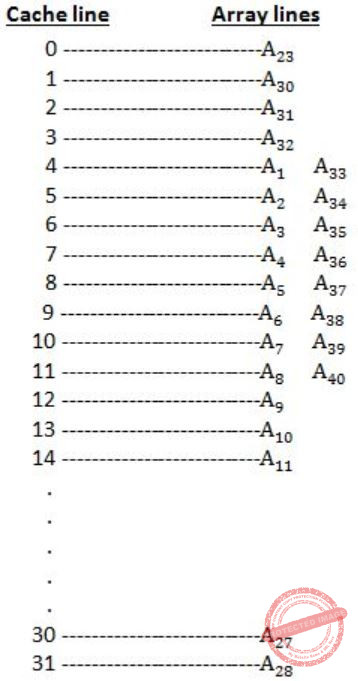So, if complete array is accessed twice, total no. of cache misses is,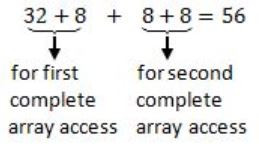Question 81

Consider a machine with a byte addressable main memory of 216 bytes. Assume that a direct mapped data cache consisting of 32 lines of 64 bytes each is used in the system. A 50 × 50 two-dimensional array of bytes is stored in the main memory starting from memory location 1100H. Assume that the data cache is initially empty. The complete array is accessed twice. Assume that the contents of the data cache do not change in between the two accesses.

Which of the following lines of the data cache will be replaced by new blocks in accessing the array for the second time?

 A line 4 to line 11 B line 4 to line 12 C line 0 to line 7 D line 0 to line 8
Computer-Organization       Cache
Question 81 Explanation:
From previous question explanation figure we can see that if array is accessed second time then from line 4 to line 11, A33 to A40 will be replaced by A1 to A8 and then A9 to A32 all array lines will be present and again that A1 to A8 will be replaced by A33 to A40.
 Question 82

A process has been allocated 3 page frames. Assume that none of the pages of the process are available in the memory initially. The process makes the following sequence of page references (reference string): 1, 2, 1, 3, 7, 4, 5, 6, 3, 1

If optimal page replacement policy is used, how many page faults occur for the above reference string?

 A 7 B 8 C 9 D 10
Operating-Systems       Page-Replacement
Question 82 Explanation:
Given sequence is
1, 2, 1, 3, 7, 4, 5, 6, 3, 1
No. of frames = 3
Using Optimal page replacement,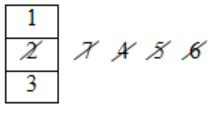Total 7 page faults.
 Question 83

A process has been allocated 3 page frames. Assume that none of the pages of the process are available in the memory initially. The process makes the following sequence of page references (reference string): 1, 2, 1, 3, 7, 4, 5, 6, 3, 1

Least Recently Used (LRU) page replacement policy is a practical approximation to optimal page replacement. For the above reference string, how many more page faults occur with LRU than with the optimal page replacement policy?

 A 0 B 1 C 2 D 3
Operating-Systems       Page-Replacement
Question 83 Explanation:
Given sequence is
1, 2, 1, 3, 7, 4, 5, 6, 3, 1 br> No. of frames = 3 (Using LRU)No. of page faults with LRU = 9
⟶ In the LRU we replace the page with least recently used page.
Using optimal page replacement
⟶ 1,2,1,3,7,4,5,6,3,1No. of page faults with optimal = 7
Difference between optimal and LRU = 9 - 7 = 2
In optimal we replace the page which will occur last in the future.
 Question 84

Suppose that a robot is placed on the Cartesian plane. At each step it is allowed to move either one unit up or one unit right, i.e., if it is at (i,j) then it can move to either (i+1,j) or (i,j+1).

How many distinct paths are there for the robot to reach the point (10,10) starting from the initial position (0,0)?

 AB 220 C 210 D None of the above
Engineering-Mathematics       Combinatorics
Question 84 Explanation:
Lets name one unit up movement as 'u' and one unit right movement as 'r'.
So now we have 10 u's and 10 r's, i.e.,
uuuuuuuuuurrrrrrrrrr
So, finally the no. of arrangements of above sequences is,Question 85

Suppose that a robot is placed on the Cartesian plane. At each step it is allowed to move either one unit up or one unit right, i.e., if it is at (i,j) then it can move to either (i+1,j) or (i,j+1).

Suppose that the robot is not allowed to traverse the line segment from (4,4) to (5,4). With this constraint, how many distinct paths are there for the robot to reach (10,10) starting from (0,0)?

 A 29 B 219 CDEngineering-Mathematics       Combinatorics
Question 85 Explanation:
So to solve this lets find the no. of paths possible if line segment from (4,4) to (5,4) is taken. And then we will subtract it from the total no. of paths possible.
So, no. of paths possible if line segment from (4,4) to (5,4) is taken is,
= paths possible from (0,0) to (4,4) * paths possible from (5,4) to (10,10)
= {uuuurrrr} * {uuuuuurrrrr}Hence, the final answer is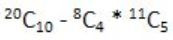There are 85 questions to complete.
PHP Code Snippets Powered By : XYZScripts.com
error: Content is protected !!